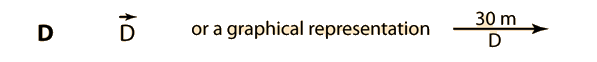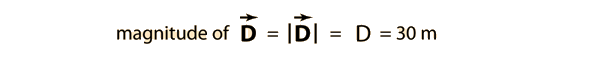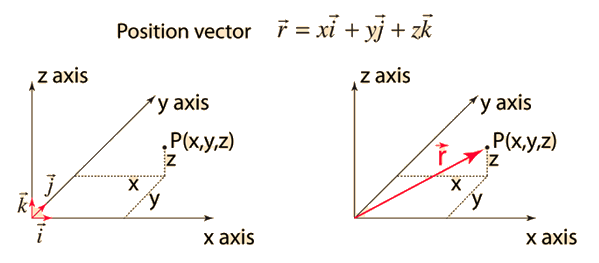# Basic Vector Definitions

A vector is a quantity that has both magnitude and direction. The magnitude is a scalar quantity, a scalar being defined as a quantity which may be completely specified by a number and perhaps a unit. Common textbook representations of vectors include boldfaced letters and boldface with an arrow above them. For example a displacement vector of 30 meters east could be represented in a variety of ways:The magnitude of the vector might be represented by absolute value signs around the vector symbol, or just the letter without the boldface.A vector might also be expressed in terms of unit vectors.

Index

Vector concepts

 HyperPhysics****HyperMath*****Vectors R Nave
Go Back

# Unit Vectors

It is convenient to use vectors of unit length to specify the directions of vector quantities in the various coordinate systems. In cartesian coordinates it is typical to use i, j and k to represent unit vectors in the x, y and z directions respectively. A vector which specifies a position in space with respect to the origin of the coordinate system could then be written.Index

Vector concepts

 HyperPhysics****HyperMath*****Vectors R Nave
Go Back